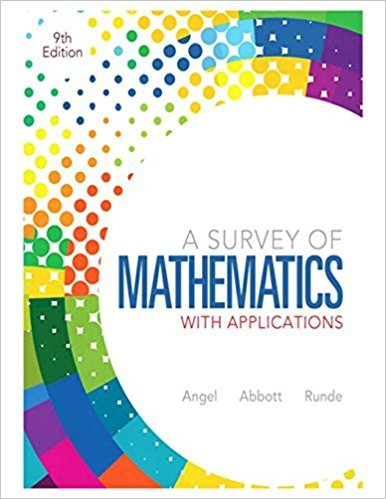×
×

# Solutions for Chapter 13: Statistics## Full solutions for A Survey of Mathematics with Applications | 9th Edition

ISBN: 9780321759665Solutions for Chapter 13: Statistics

Solutions for Chapter 13
4 5 0 292 Reviews
16
0
##### ISBN: 9780321759665

This textbook survival guide was created for the textbook: A Survey of Mathematics with Applications, edition: 9. This expansive textbook survival guide covers the following chapters and their solutions. Chapter 13: Statistics includes 72 full step-by-step solutions. Since 72 problems in chapter 13: Statistics have been answered, more than 80235 students have viewed full step-by-step solutions from this chapter. A Survey of Mathematics with Applications was written by and is associated to the ISBN: 9780321759665.

Key Math Terms and definitions covered in this textbook
• Characteristic equation det(A - AI) = O.

The n roots are the eigenvalues of A.

A sequence of steps (end of Chapter 9) to solve positive definite Ax = b by minimizing !x T Ax - x Tb over growing Krylov subspaces.

• Gauss-Jordan method.

Invert A by row operations on [A I] to reach [I A-I].

• Graph G.

Set of n nodes connected pairwise by m edges. A complete graph has all n(n - 1)/2 edges between nodes. A tree has only n - 1 edges and no closed loops.

• Hankel matrix H.

Constant along each antidiagonal; hij depends on i + j.

• Jordan form 1 = M- 1 AM.

If A has s independent eigenvectors, its "generalized" eigenvector matrix M gives 1 = diag(lt, ... , 1s). The block his Akh +Nk where Nk has 1 's on diagonall. Each block has one eigenvalue Ak and one eigenvector.

• Minimal polynomial of A.

The lowest degree polynomial with meA) = zero matrix. This is peA) = det(A - AI) if no eigenvalues are repeated; always meA) divides peA).

• Multiplication Ax

= Xl (column 1) + ... + xn(column n) = combination of columns.

• Multiplier eij.

The pivot row j is multiplied by eij and subtracted from row i to eliminate the i, j entry: eij = (entry to eliminate) / (jth pivot).

• Network.

A directed graph that has constants Cl, ... , Cm associated with the edges.

• Pivot.

The diagonal entry (first nonzero) at the time when a row is used in elimination.

• Semidefinite matrix A.

(Positive) semidefinite: all x T Ax > 0, all A > 0; A = any RT R.

• Similar matrices A and B.

Every B = M-I AM has the same eigenvalues as A.

• Solvable system Ax = b.

The right side b is in the column space of A.

• Stiffness matrix

If x gives the movements of the nodes, K x gives the internal forces. K = ATe A where C has spring constants from Hooke's Law and Ax = stretching.

• Toeplitz matrix.

Constant down each diagonal = time-invariant (shift-invariant) filter.

• Triangle inequality II u + v II < II u II + II v II.

For matrix norms II A + B II < II A II + II B II·

v + w = (VI + WI, ... , Vn + Wn ) = diagonal of parallelogram.

• Vector v in Rn.

Sequence of n real numbers v = (VI, ... , Vn) = point in Rn.

• Volume of box.

The rows (or the columns) of A generate a box with volume I det(A) I.

×